# Grids and graticules in the tmap package

vignette
Author

Published

September 4, 2019

This vignette builds on the making maps chapter of the Geocomputation with R book. Its goal is to demonstrate how to set and modify grids and graticules in the tmap package.

## Prerequisites

The examples below assume the following packages are attached:

``````library(spData) # example datasets
library(tmap)   # map creation (>=2.3)
library(sf)     # spatial data classes``````

# Grids and graticules

The tmap package offers two ways to draws coordinate lines - `tm_grid()` and `tm_graticules()`. The role of `tm_grid()` is to represent the input data’s coordinates. For example, the `nz` object uses the New Zealand Transverse Mercator projection, with meters as its units.

``````tm_shape(nz) +
tm_polygons() +
tm_grid()``````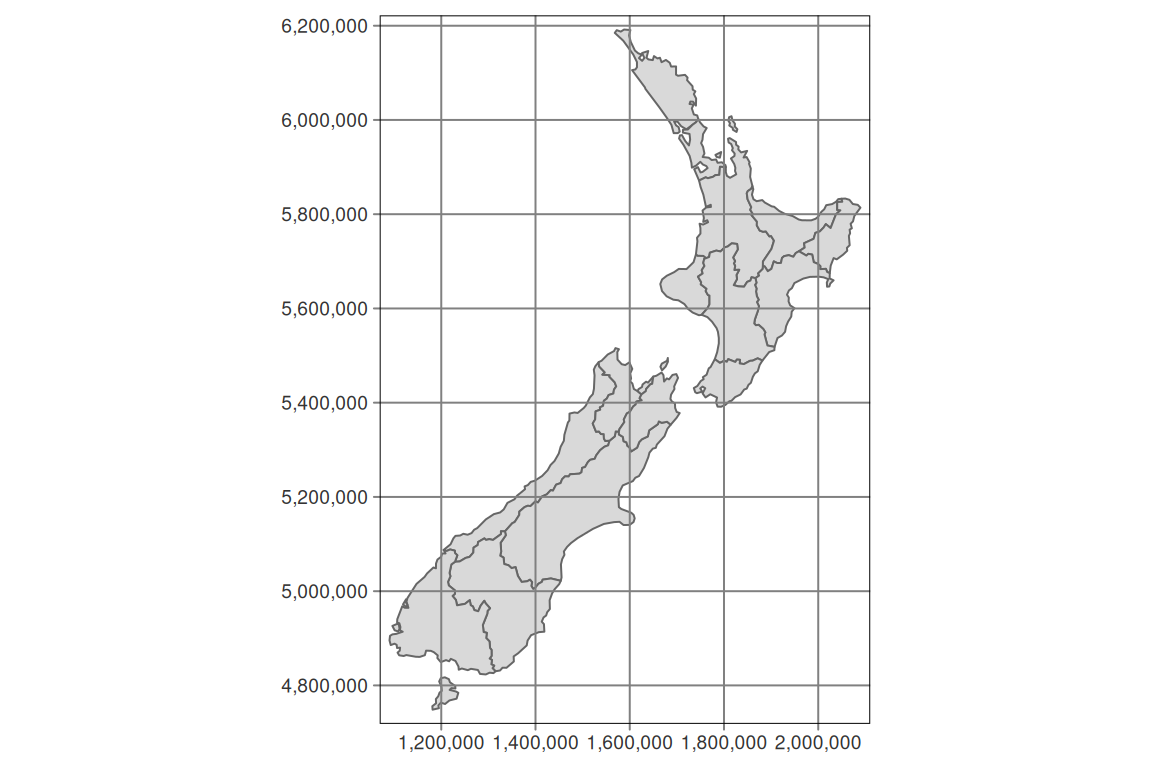`tm_graticules()` shows longitude lines (meridians) and latitude lines (parallels), with degrees as units (note the degree sign in the example below).

``````tm_shape(nz) +
tm_polygons() +
tm_graticules()``````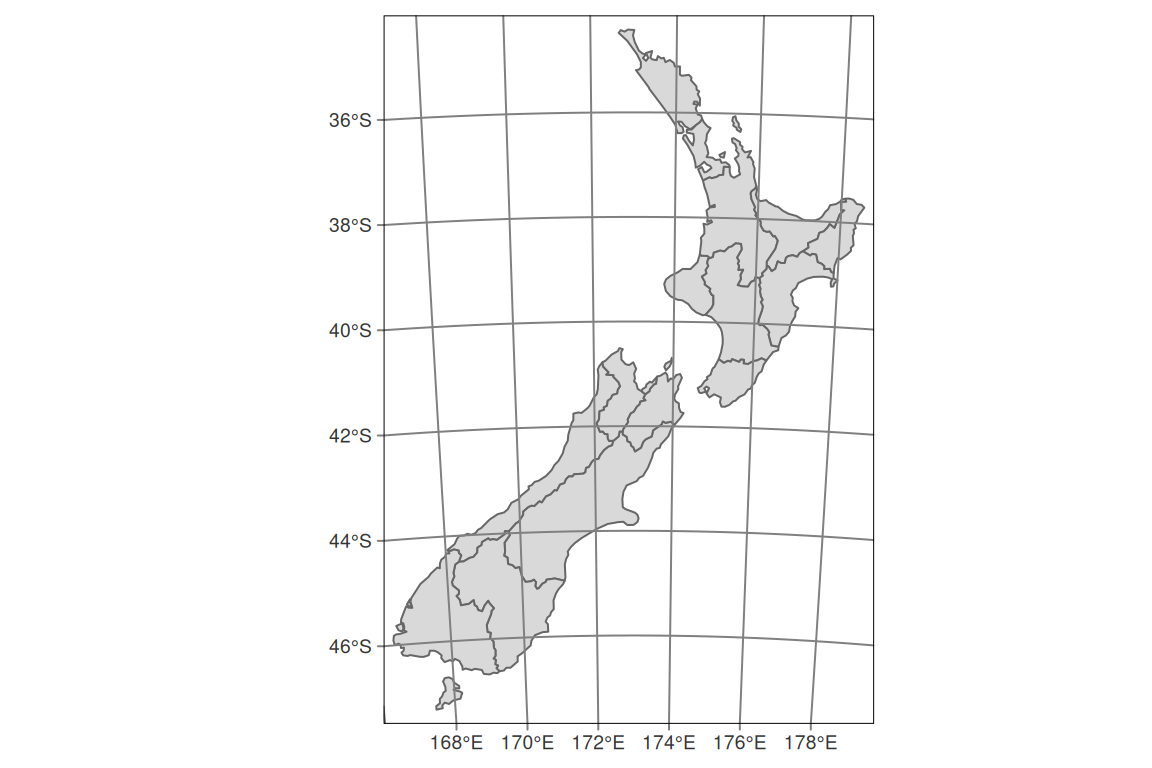# Layers order

Both, `tm_grid()` and `tm_graticules()` could be placed above or below the main spatial data. Its position on the map depends on its place in the code. When `tm_grid()` or `tm_graticules()` are placed after the code drawing geometry (e.g. `tm_polygons()`), the grids or graticules are ploted on the top of the map. On the other hand, when `tm_grid()` or `tm_graticules()` are placed before the code drawing geometry (e.g. `tm_polygons()`), the grids or graticules are plotted behind the spatial data.

``````tm_shape(nz) +
tm_graticules() +
tm_polygons()``````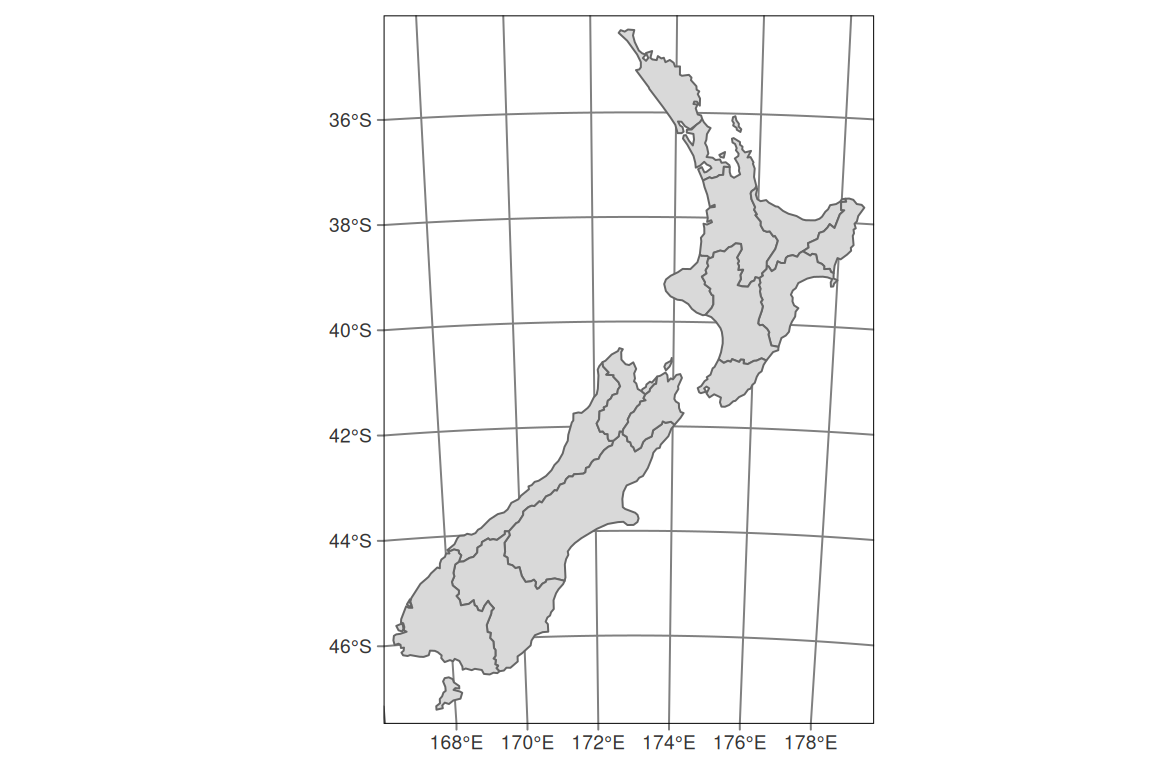# Customization

Grids and graticules can be easily customized in tmap using several arguments. The first one, `labels.inside.frame` moves the labels inside the map grid (it is set to `FALSE` as the default).

``````tm_shape(nz) +
tm_grid(labels.inside.frame = TRUE) +
tm_polygons()``````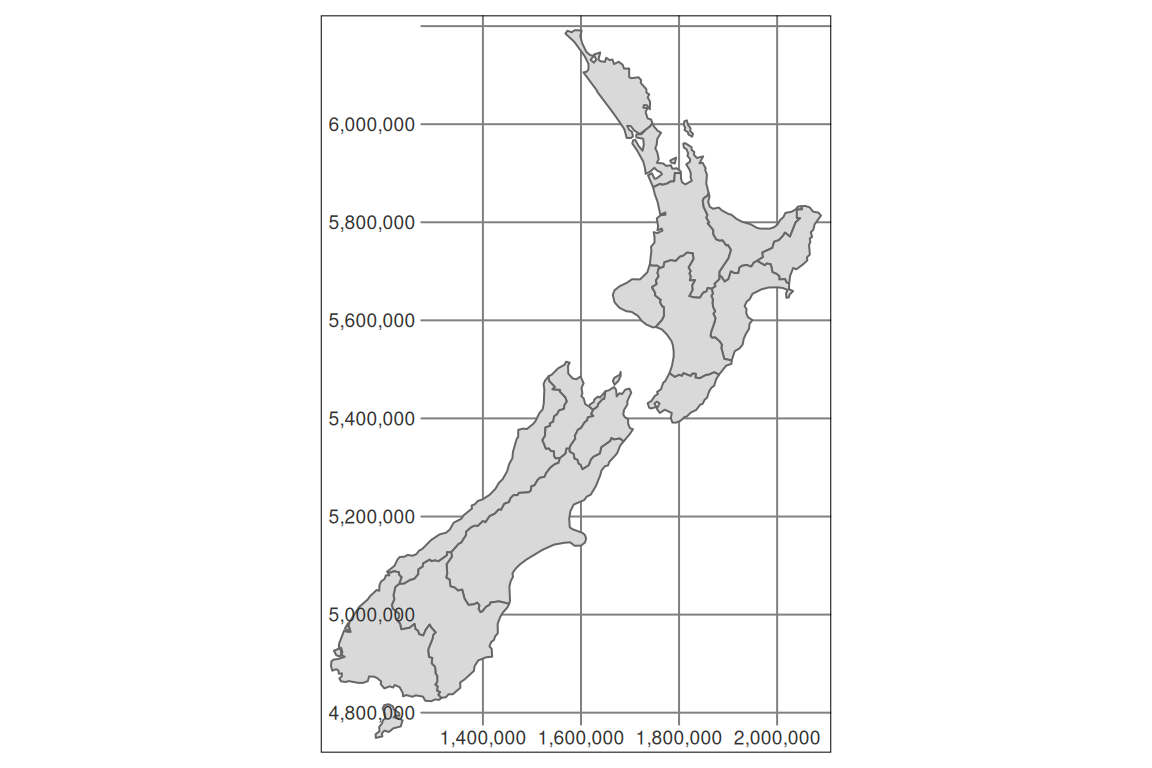The number of horizontal (`x`) and vertical (`y`) lines can be set using the `n.x` and `n.y` arguments. Importantly, tmap rounds coordinate values to equally spaced “round” values, so the number of actual labels may be slightly different than set with `n.x` and `n.y`.

``````tm_shape(nz) +
tm_grid(n.x = 4, n.y = 3) +
tm_polygons()``````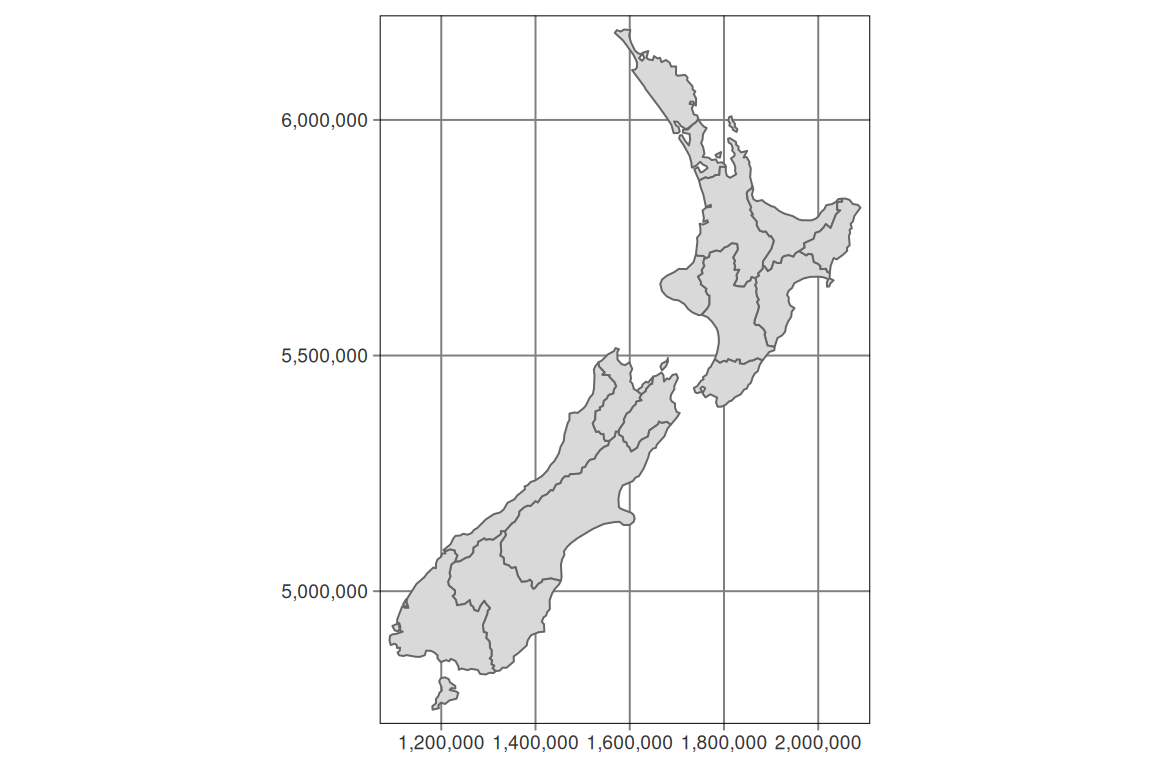By default, `tm_grid()` and `tm_graticules()` shows ticks and lines. They can be disabled using `ticks = FALSE` and `lines = FALSE`.

``````tm_shape(nz) +
tm_grid(ticks = FALSE) +
tm_polygons()``````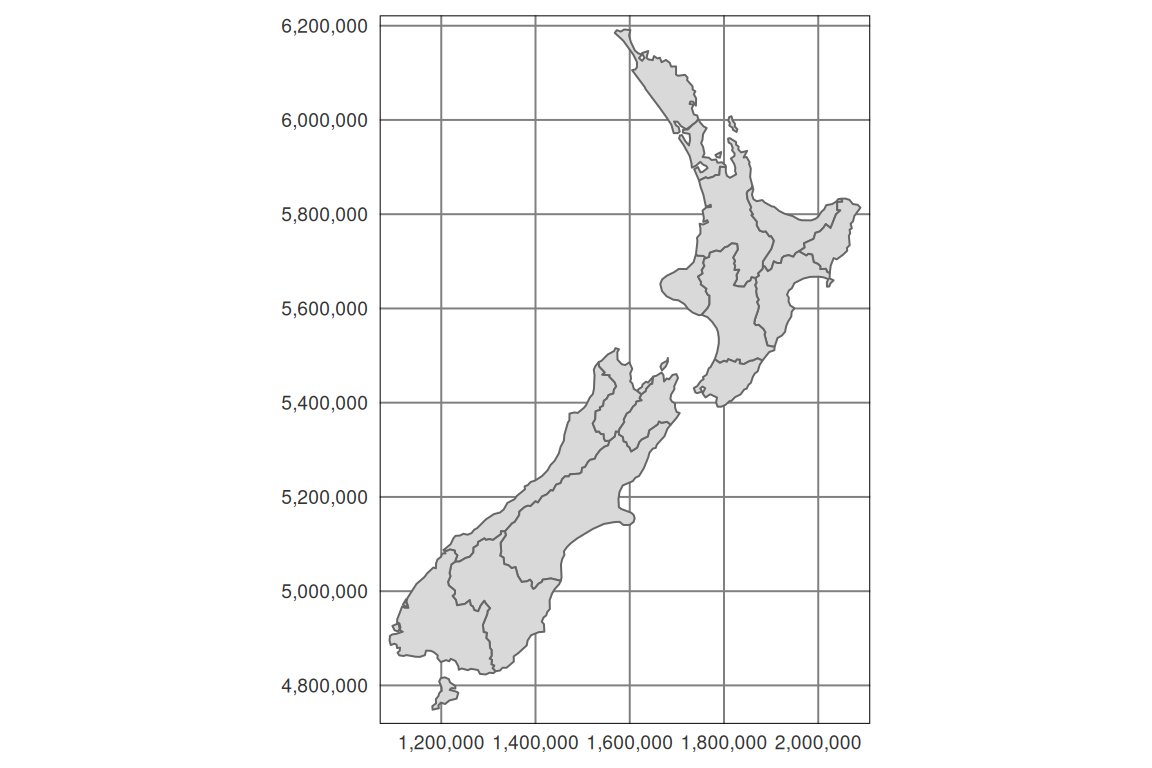Especially, `lines = FALSE` could be useful when presenting raster data.

``````tm_shape(nz) +
tm_grid(lines = FALSE) +
tm_polygons()``````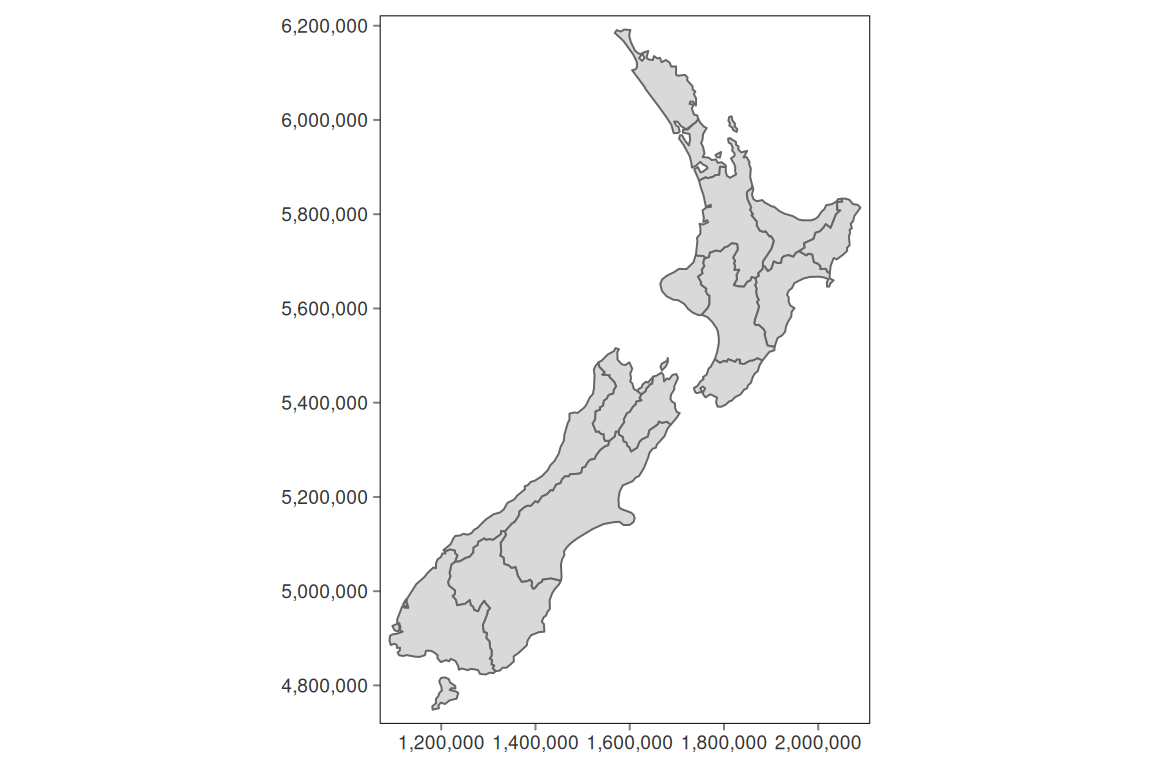It is also possible to customize `tm_grid()` and `tm_graticules()` apperance, for example by chaning the lines colors (`col`), width (`lwd`) or labels size (`labels.size`).

``````tm_shape(nz) +
tm_grid(col = "red", lwd = 3, labels.size = 0.4) +
tm_polygons()``````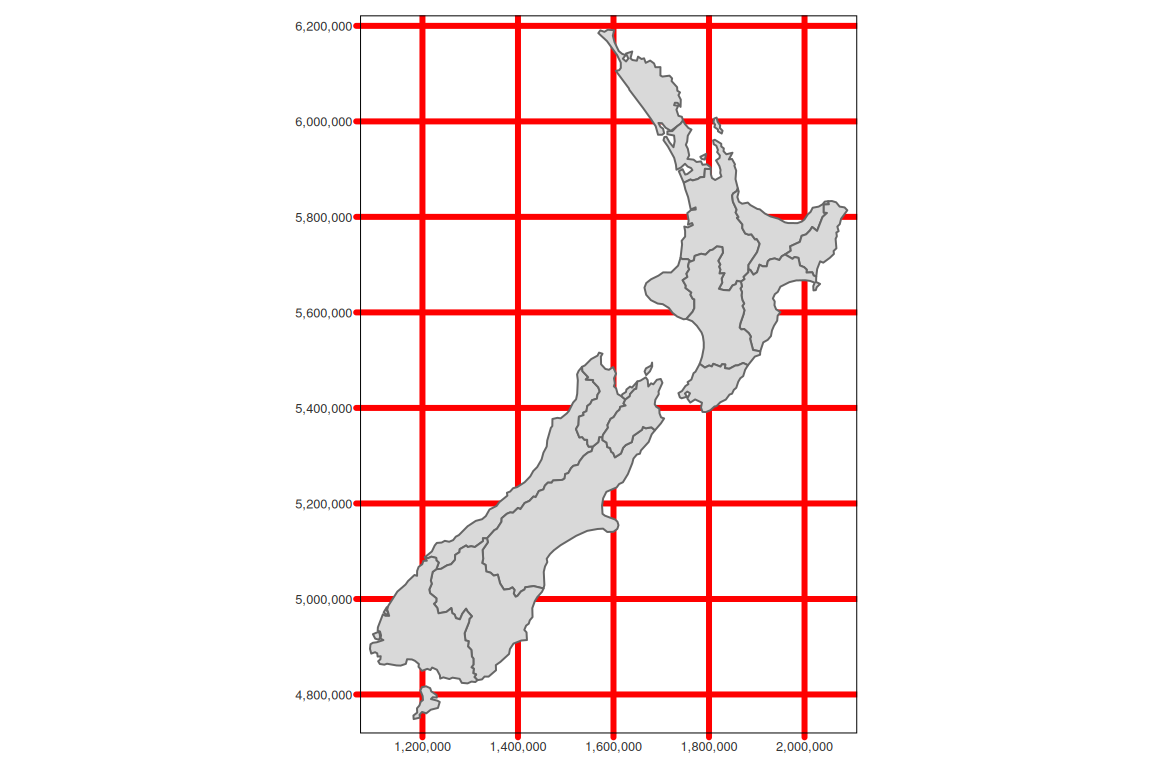The above examples uses `tm_grid()`, but the same arguments apply to the `tm_graticules()`.

# Layout settings

By default, tmap adds small inner margins between the presented data and the map frame. It works well in many cases, for example, see the map of New Zealand above. However, it does not look perfect for world maps.

``````tm_shape(world) +
tm_graticules() +
tm_polygons()``````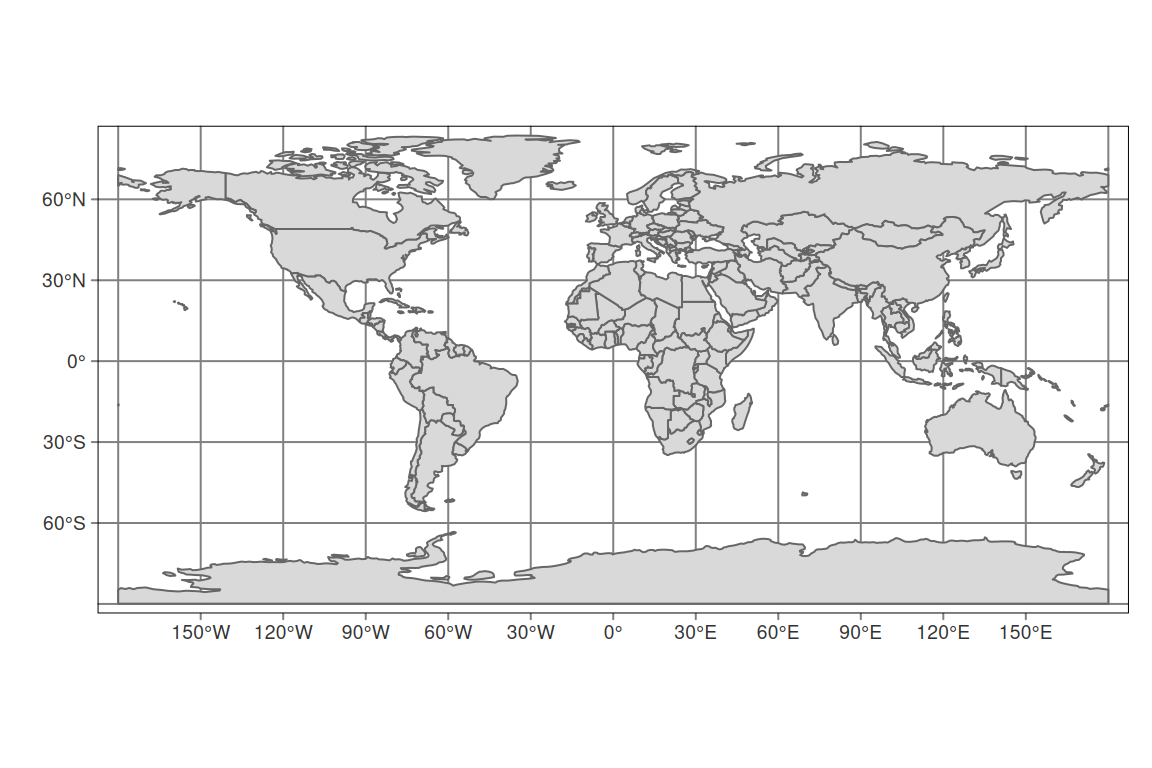The way to fix this is to use the `tm_layout()` function and set its `inner.margins` argument to `0`.

``````tm_shape(world) +
tm_graticules() +
tm_polygons() +
tm_layout(inner.margins = 0)``````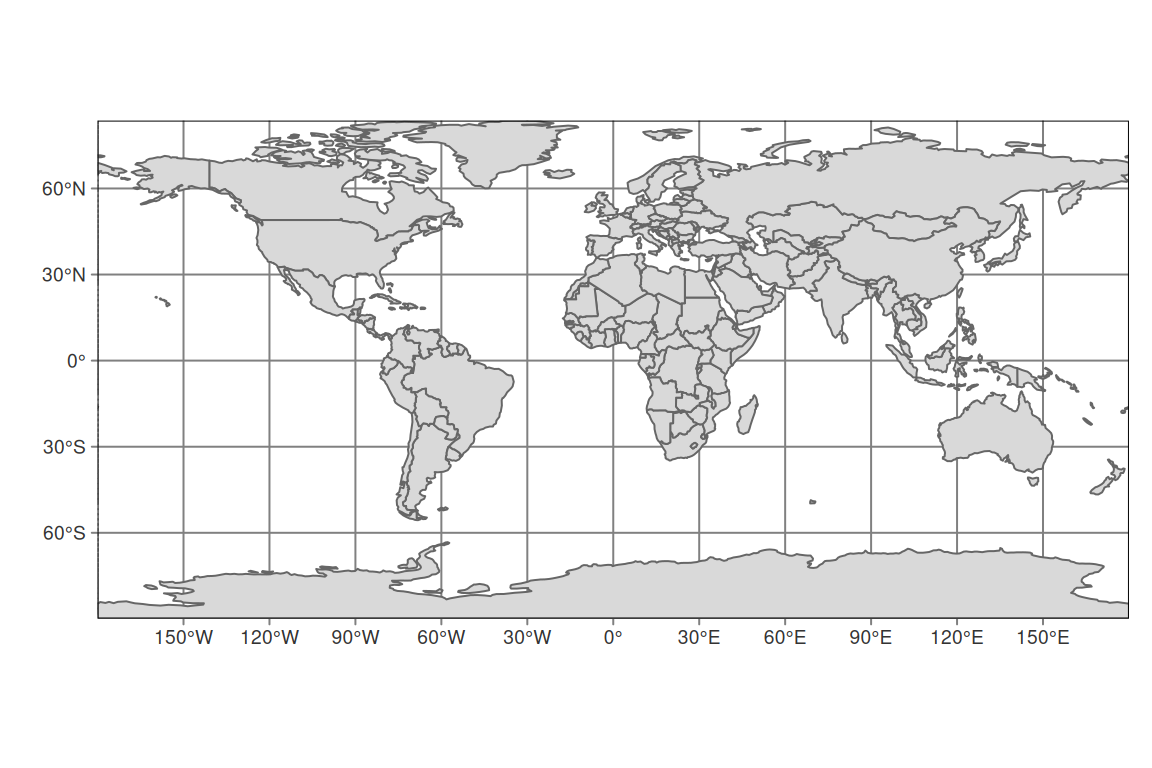## Citation

BibTeX citation:
``````@online{nowosad2019,